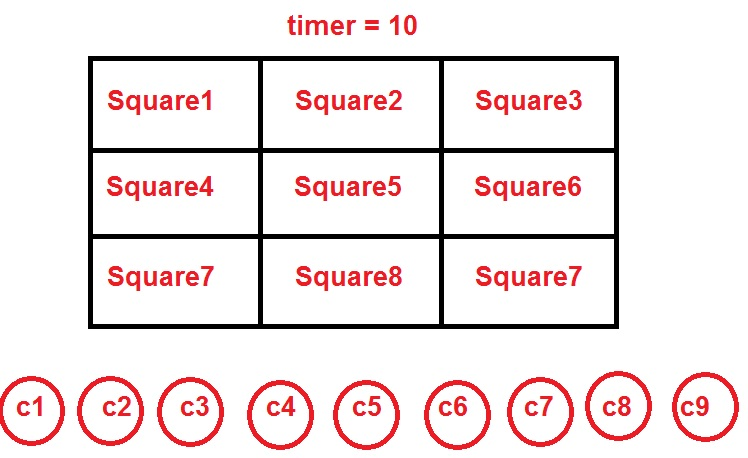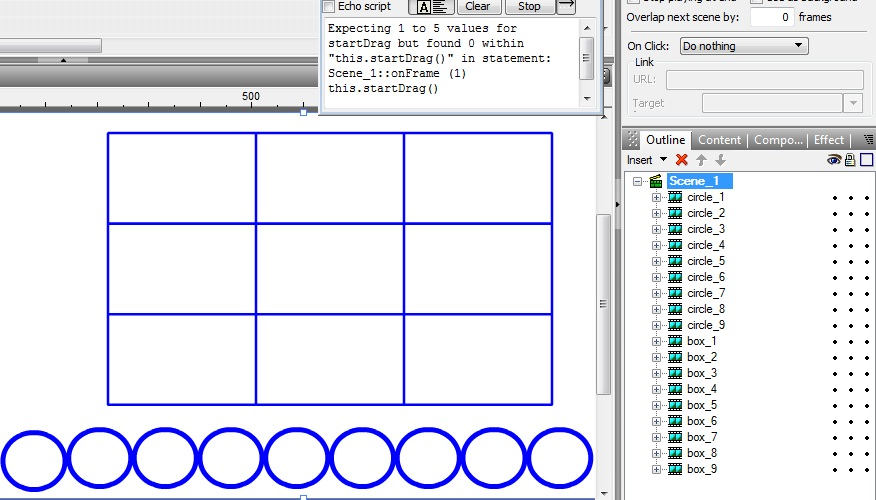A Flash Developer Resource Site

# Thread: drag and drop need help actionscript 2

1. ## drag and drop need help actionscript 2

hello all
excuse me for my bad English

i have 9 squares and 9 circlesi need :
if i click square1 ===> drag any of circles and drop into square1 only after that:

1- stop drag and drop this circle

1- stop drag and drop into this square1

2- stop square1 press

and so on if i click square2 ===> drag any of circles and drop into square2 only and so on

What is the simplest code to do this

my code is :

PHP Code:
``` on (press) { if(timer >0){startDragLocked(); } onSelfEvent (release,releaseOutside) {    stopDrag();     }  ```
thanks for helpReply With Quote

2. Alright so this is the script for your main timeline.

circle_array is for all the circle movieclip names and amounts
box_array is for all the box movieclip names and amounts

the stage just needs circles and boxes with instance names circle_1 etc.. box_1 etc..
PHP Code:
``` var current_selected=null var drag_mode_enabled=false var box_available=null var circle_array=[circle_1,circle_2,circle_3,circle_4,circle_5,circle_6,circle_7,circle_8,circle_9] var box_array=[box_1,box_2,box_3,box_4,box_5,box_6,box_7,box_8,box_9] for(var i=0;i<circle_array.length;i++){ circle_array[i].startX=circle_array[i]._x circle_array[i].startY=circle_array[i]._y } for(var m=0;m<box_array.length;m++){ box_array[m].taken=false box_array[m].num=m box_array[m].onPress=function(){ if(this.taken==false){ drag_mode_enabled=true box_available=this.num } } } for(var j=0;j<circle_array.length;j++){ circle_array[j].onPress=function(){ if(drag_mode_enabled){ this.atBox=false this.startDrag() } } circle_array[j].onRelease=function(){ for(var k=0;k<box_array.length;k++){ if(this.atBox==false&&distanceChecker(this._x,box_array[k]._x)<=37&&distanceChecker(this._y,box_array[k]._y)<=37&&box_array[k].taken==false&&box_available==k){ this.stopDrag() box_array[k].taken=true this._x=box_array[k]._x this._y=box_array[k]._y drag_mode_enabled=false this.atBox=true this._alpha=50 }else if(!this.atBox&&k==box_array.length-1){ this._alpha=100 this._x=this.startX this._y=this.startY this.stopDrag() drag_mode_enabled=false } } } } function distanceChecker(a,b){ if(a>b){ return(a-b) }else{ return(b-a); } }  ```Reply With Quote

3. This main timeline script below gives you more chances to let go of the circle if it hasn't locked to the box yet:

The boxes should have a lower depth than the circles on the stage.

The registration point for the boxes and circles should be centered for the distanceChecker function to detect where you drop the circle

PHP Code:
``` var current_selected=null var drag_mode_enabled=false var box_available=null var circle_array=[circle_1,circle_2,circle_3,circle_4,circle_5,circle_6,circle_7,circle_8,circle_9] var box_array=[box_1,box_2,box_3,box_4,box_5,box_6,box_7,box_8,box_9] for(var i=0;i<circle_array.length;i++){ circle_array[i].startX=circle_array[i]._x circle_array[i].startY=circle_array[i]._y } for(var m=0;m<box_array.length;m++){ box_array[m].taken=false box_array[m].num=m box_array[m].onPress=function(){ if(this.taken==false){ drag_mode_enabled=true box_available=this.num } } } for(var j=0;j<circle_array.length;j++){ circle_array[j].onPress=function(){ if(drag_mode_enabled){ this.atBox=false this.startDrag() } } circle_array[j].onRelease=function(){ for(var k=0;k<box_array.length;k++){ if(this.atBox==false&&distanceChecker(this._x,box_array[k]._x)<=37&&distanceChecker(this._y,box_array[k]._y)<=37&&box_array[k].taken==false&&box_available==k){ this.stopDrag() box_array[k].taken=true this._x=box_array[k]._x this._y=box_array[k]._y drag_mode_enabled=false this.atBox=true this._alpha=50 }else if(!this.atBox&&k==box_array.length-1){ this._alpha=100 this._x=this.startX this._y=this.startY this.stopDrag() } } } } function distanceChecker(a,b){ if(a>b){ return(a-b) }else{ return(b-a); } }  ```Reply With Quote

4. hello Alloy Bacon

i have this error in my swishmax

HTML Code:
```Expecting 1 to 5 values for startDrag but found 0 within "this.startDrag()" in statement:
Scene_1::onFrame (1)
this.startDrag()```Reply With Quote

5. This is for as2, I dont use swishmax,

try adding a 5 for those errors inside the ()

this lets you drag from center or from where you clicked it based on 0-5
PHP Code:
``` this.startDrag(5)  ```Reply With Quote

6. oh I left 37 as the distance to snap, heres a fix:

might work for swishmax
PHP Code:
``` var current_selected=null var drag_mode_enabled=false var box_available=null var circle_array=[circle_1,circle_2,circle_3,circle_4,circle_5,circle_6,circle_7,circle_8,circle_9] var box_array=[box_1,box_2,box_3,box_4,box_5,box_6,box_7,box_8,box_9] for(var i=0;i<circle_array.length;i++){ circle_array[i].startX=circle_array[i]._x circle_array[i].startY=circle_array[i]._y } for(var m=0;m<box_array.length;m++){ box_array[m].taken=false box_array[m].num=m box_array[m].onPress=function(){ if(this.taken==false){ drag_mode_enabled=true box_available=this.num } } } for(var j=0;j<circle_array.length;j++){ circle_array[j].onPress=function(){ if(drag_mode_enabled){ this.atBox=false this.startDrag(5) } } circle_array[j].onRelease=function(){ for(var k=0;k<box_array.length;k++){ trace(box_array._width) if(this.atBox==false&&distanceChecker(this._x,box_array[k]._x)<=box_array._width&&distanceChecker(this._y,box_array[k]._y)<=box_array._height&&box_array[k].taken==false&&box_available==k){ this.stopDrag(5) box_array[k].taken=true this._x=box_array[k]._x this._y=box_array[k]._y drag_mode_enabled=false this.atBox=true this._alpha=50 }else if(!this.atBox&&k==box_array.length-1){ this._alpha=100 this._x=this.startX this._y=this.startY this.stopDrag(5) } } } } function distanceChecker(a,b){ if(a>b){ return(a-b) }else{ return(b-a); } }  ```Reply With Quote

7. no error found but the circle not drag (not moving)Reply With Quote

8. You told me that the box must be clicked first.

instructions:
1.)click a box
2.) drag any circle specifically to the clicked boxReply With Quote

9. hello Alloy Bacon
thank you very much
its working now
thanksReply With Quote

10. hello hello Alloy Bacon
there is a problem:
for example
when i press box_1 and drop circle_1 this is ok but when i press box_2 and drag and drop in box_1 again the circle droped again into box_1

i want disable droped into box when droped befor

thanks for helpReply With Quote

11. For as2 its working fine, I dont have access to swishmax the download needs a keyReply With Quote

12. this is swishmax link

https://app.box.com/s/qgdzjsdhsv2bg3k4izneag6sybmocnl3Reply With Quote

13. Ok I am testing it, I found the problem.Reply With Quote

14. Ok so now
1) .enabled=false //when the circles at the box
2) .swapDepths(300) //the circle gets highest depth when dragged
3) .onReleaseOutside // so the circle doesnt follow the mouse if it is released
4) //nice graphics kofa

PHP Code:
``` onFrame (1) {     stop();     var drag_mode_enabled=false var box_available=null var circle_array=[circle_1,circle_2,circle_3,circle_4,circle_5,circle_6,circle_7,circle_8,circle_9] var box_array=[box_1,box_2,box_3,box_4,box_5,box_6,box_7,box_8,box_9] for(var i=0;i<circle_array.length;i++){ circle_array[i].startX=circle_array[i]._x circle_array[i].startY=circle_array[i]._y } for(var m=0;m<box_array.length;m++){ box_array[m].taken=false box_array[m].num=m box_array[m].onPress=function(){ if(this.taken==false){ drag_mode_enabled=true box_available=this.num } } } for(var j=0;j<circle_array.length;j++){ circle_array[j].onPress=function(){ if(drag_mode_enabled){ this.atBox=false this.swapDepths(300) this.startDrag(5) } } circle_array[j].onRelease=circle_array[j].onReleaseOutside=function(){ for(var k=0;k<box_array.length;k++){ trace(box_array._width) if(this.atBox==false&&distanceChecker(this._x,box_array[k]._x)<=box_array._width&&distanceChecker(this._y,box_array[k]._y)<=box_array._height&&box_array[k].taken==false&&box_available==k){ this.stopDrag(5) this.enabled=false box_array[k].taken=true this._x=box_array[k]._x this._y=box_array[k]._y drag_mode_enabled=false this.atBox=true this._alpha=50 }else if(!this.atBox&&k==box_array.length-1){ this._alpha=100 this._x=this.startX this._y=this.startY this.stopDrag(5) } } } } function distanceChecker(a,b){ if(a>b){ return(a-b) }else{ return(b-a); } }  }  ```Reply With Quote

15. hello Alloy Bacon
i am very sorry
there is a new problem
when i press box_1 and drag and drop into box_2 , the circle dropped in box_1
i want if i press box_1 and the circle near box_1 only (move to box_1 only) else return back
excuse me for my bad englishReply With Quote

16. You can limit the snap on bounds by modifying the distance checker statement: /2 now

If this does not solve the issue, can you post a screen record?

PHP Code:
``` var current_selected=null var drag_mode_enabled=false var box_available=null var circle_array=[circle_1,circle_2,circle_3,circle_4,circle_5,circle_6,circle_7,circle_8,circle_9] var box_array=[box_1,box_2,box_3,box_4,box_5,box_6,box_7,box_8,box_9] for(var i=0;i<circle_array.length;i++){ circle_array[i].startX=circle_array[i]._x circle_array[i].startY=circle_array[i]._y } for(var m=0;m<box_array.length;m++){ box_array[m].taken=false box_array[m].num=m box_array[m].onPress=function(){ if(this.taken==false){ drag_mode_enabled=true box_available=this.num } } } for(var j=0;j<circle_array.length;j++){ circle_array[j].onPress=function(){ if(drag_mode_enabled){     this.swapDepths(300) this.atBox=false this.startDrag(5) } } circle_array[j].onRelease=circle_array[j].onReleaseOutside=function(){ for(var k=0;k<box_array.length;k++){ trace(box_array._width) if(this.atBox==false&&distanceChecker(this._x,box_array[k]._x)<=box_array._width/2&&distanceChecker(this._y,box_array[k]._y)<=box_array._height/2&&box_array[k].taken==false&&box_available==k){ this.stopDrag(5) this.enabled=false box_array[k].taken=true this._x=box_array[k]._x this._y=box_array[k]._y drag_mode_enabled=false this.atBox=true this._alpha=50 }else if(!this.atBox&&k==box_array.length-1){ this._alpha=100 this._x=this.startX this._y=this.startY this.stopDrag(5) } } } } function distanceChecker(a,b){ if(a>b){ return(a-b) }else{ return(b-a); } }  ```Reply With Quote

17. thank you very very much my friend

it is working fine now

you are a good man

thanks for help

happy timeReply With Quote

18. hello Alloy Bacon
if you have a free time can you add some features
can you create a textfield into each boxes which loads rondom text from array for example

on frame(1)
this.create (9 )text field
my_array = [ text1 , text2 , text3.........]
text field_1=my_array 
text field_2=my_array 

when the circle droped into box , the circle create text field = the same text in box

excuse me again for my bad englishReply With Quote

19. The box movieclip needs a text field with an instance name of: data_field

The circle movieclip needs a text field with an instance name of: data_field

PHP Code:
``` var current_selected=null var drag_mode_enabled=false var box_available=null var circle_array=[circle_1,circle_2,circle_3,circle_4,circle_5,circle_6,circle_7,circle_8,circle_9] var box_array=[box_1,box_2,box_3,box_4,box_5,box_6,box_7,box_8,box_9] var text_array=['txt1','txt2','txt3','txt4','txt5','txt6','txt7','txt8','txt9'] for(var i=0;i<circle_array.length;i++){ circle_array[i].startX=circle_array[i]._x circle_array[i].startY=circle_array[i]._y } for(var m=0;m<box_array.length;m++){ box_array[m].taken=false box_array[m].num=m box_array[m].data_field.text=text_array[m] box_array[m].onPress=function(){ if(this.taken==false){ drag_mode_enabled=true box_available=this.num } } } for(var j=0;j<circle_array.length;j++){ circle_array[j].onPress=function(){ if(drag_mode_enabled){     this.swapDepths(300) this.atBox=false this.startDrag(5) } } circle_array[j].onRelease=circle_array[j].onReleaseOutside=function(){ for(var k=0;k<box_array.length;k++){ trace(box_array._width) if(this.atBox==false&&distanceChecker(this._x,box_array[k]._x)<=box_array._width/2&&distanceChecker(this._y,box_array[k]._y)<=box_array._height/2&&box_array[k].taken==false&&box_available==k){ this.stopDrag(5) this.enabled=false this.data_field.text=box_array[k].data_field.text box_array[k].taken=true this._x=box_array[k]._x this._y=box_array[k]._y drag_mode_enabled=false this.atBox=true this._alpha=50 }else if(!this.atBox&&k==box_array.length-1){ this._alpha=100 this._x=this.startX this._y=this.startY this.stopDrag(5) } } } } function distanceChecker(a,b){ if(a>b){ return(a-b) }else{ return(b-a); } }  ```Reply With Quote

20. thank you Alloy Bacon

it is working fine

thanks for helpReply With Quote

####Posting Permissions

• You may not post new threads
• You may not post replies
• You may not post attachments
• You may not edit your posts
•

 » Home » Movies » Tutorials » Submissions » Board » Links » Reviews » Feedback » Gallery » Fonts » The Lounge » Sound Loops » Sound FX » About FK » Sitemap

Click Here to Expand Forum to Full Width